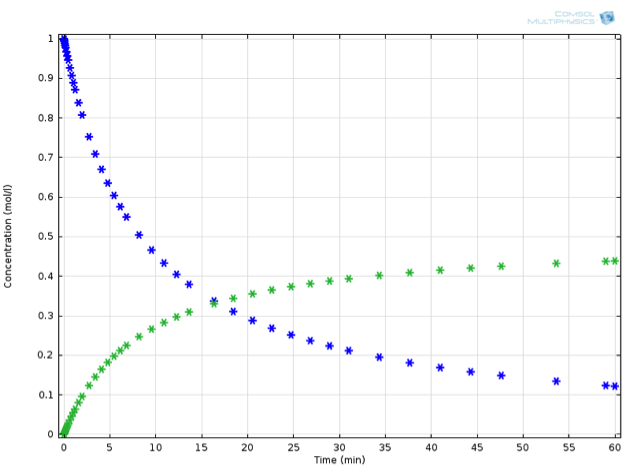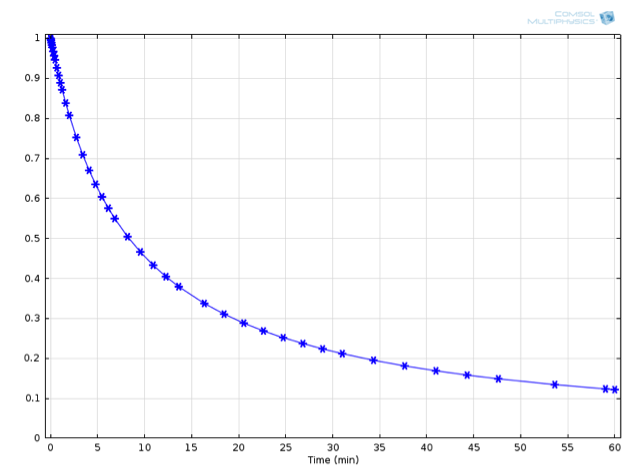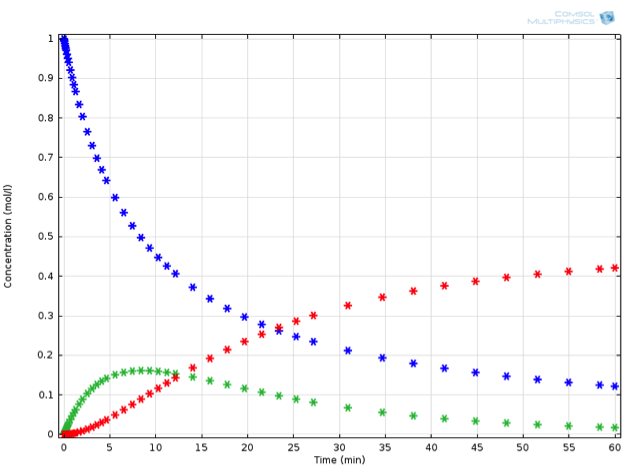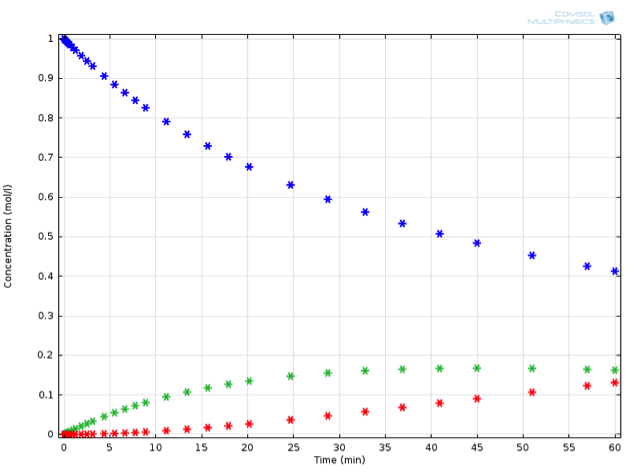# A General Introduction to Chemical Kinetics, Arrhenius Law

February 13, 2014

Chemical reaction engineering is an interesting modeling challenge. At first glance, describing a reacting system seems to be very manageable. There remain, however, countless complications and pitfalls that make chemical simulations both challenging and rewarding. In this first post of a new blog series, we will introduce chemical kinetics in general and walk you through how you can use COMSOL software in chemical reaction engineering.

### Introduction to Chemical Kinetics

Chemistry is, quite literally, vital. Any biological system relies on chemical reactions to function. Anytime we want to influence biological systems — be it to brew beer or to cure a (possibly beer-related…) headache using painkillers — we are actually influencing chemistry. But chemistry is not merely the underlying principle of biology: it is also vital to any aspect of our modern age. From solvents, colors, fuels, and lacquers to plastics and pesticides, there are more than 70,000 industrially-produced chemical compounds. Therefore, it is no surprise that the chemical industry is one of the largest contributors to the global economy. The total value of products of the chemical industry is estimated to be almost 5 trillion USD per annum! This corresponds to around 7% of the gross world product.

### A Simple Chemical Reaction Example

Take, for example, the transformation of some substance \mathrm{A} (called a reactant, substrate, or feedstock) into some other, ideally more valuable, substance (called a product) symbolically represented by the reaction equation:

2\mathrm{A} \rightarrow \mathrm{B}

Even though this equation makes no statement on the mechanism of the reaction (that is, in what order bonds are broken and formed, and which intermediate chemical species are transiently created), it still allows us to mathematically describe the macroscopic rate of reaction. To better understand the concept of a macroscopic reaction rate, imagine a virtual experiment where we allow substance A to react in a beaker and intermittently take a sample with a fixed volume. We then place this sample into an analytic device that, through some means, can count the number of particles of \mathrm{A} and \mathrm{B} that are present in the sample. By measuring the number of particles and dividing it by the sample volume, we are in fact measuring a concentration.

Concentrations are usually described in the units of mol/L or mol/m3. A mole is a unit of “amount of substance”, and is a shorthand for N_A atoms, molecules, electrons, or whatever we are measuring. This is convenient because the number of molecules occurring in practical samples is very large; Avogadro’s number N_A represents a very large number of molecules (roughly 6×1023/mol).

Returning to our virtual experiment, we might observe behavior according to the plot below:Concentration profile in time: Substance A (blue) decreases as substance B (green) increases. Note that the decrease of A is twice as fast as the increase of B.

Can we propose a mathematical expression to describe the rate of the chemical reaction? As the concentration of the reactant increases, it is likely that the rate of reaction will also increase, but with what proportionality? One suggestion is the law of mass action, which imagines reactions due to collisions between reactants. We propose that the rate at which \mathrm{A} reacts is proportional to the likelihood of two particles of \mathrm{A} meeting each other. This suggests the following fundamental rate law for the examined reaction:

r_1=k_1\cdot{c_\mathrm{A}^2}

where k_1 is called the reaction rate constant (we will qualify the use of the term “constant” below, but for now it may be assumed). This is called a “second-order” reaction in the reactant \mathrm{A} since the rate is proportional to the second power of the reactant concentration: that proportionality arises from the collisional argument above. It is important to emphasize that the law of mass action does not apply to all chemical reactions; as discussed above, the observed rate law does not correlate directly with the actual mechanism of the reaction.

In any rate law, the units of the rate constant k depend on the reaction order: in this case k_1 has the unit of (L/mol)/s (pronounced “per molar per second”). We can now use this expression for r_1 to determine the change in concentration for \mathrm{A} and \mathrm{B}:

\begin{split}
\frac{dc_\mathrm{A}}{dt} %26= -2{r_1}\\
\frac{dc_\mathrm{B}}{dt} %26= +r_1
\end{split}

Note the multipliers -2 and +1 in the respective equations for \mathrm{A} and \mathrm{B}. This is due to the consumption of two particles of \mathrm{A} for the production of one particle of \mathrm{B}. The multipliers are called stoichiometric coefficients or stoichiometric numbers, and they are often written with the Greek letter nu (\nu).

The chemical rate law yields a set of two ordinary differential equations (ODEs), which are intrinsically simpler to solve than the usual space-dependent partial differential equations (PDEs) that COMSOL Multiphysics users encounter. The equation set for the reaction system described above can easily be integrated to obtain:

{c_\mathrm{A}} = \frac{c_\mathrm{A,0}}{1 + 2c_\mathrm{A,0}\cdot{k_1} {t}}

Here, c_\mathrm{A,0} represents the initial concentration of species \mathrm{A} in the reaction vessel. This expression is graphically shown below alongside the numerically derived values for the concentration:Numerically (asterisks) and analytically (solid line) derived concentrations of A over time.

For this example, an initial concentration for \mathrm{A} of 1 mol/L was used, as well as a value for {k_1} of 0.001 (L/mol)/s at 20°C.

### A Spanner in the Works

In general, the chemical rate expressions will be a combination of terms, in this form:

r_i = k_i \prod_j{c_j^{\nu_{ij}}}

for the reaction, i, of a set of chemical species, j, with respective reaction orders, \nu_{ij}. Again, the reaction order will often correspond to the stoichiometry, but for some reaction mechanisms this is not necessarily the case.

It is apparent that these expressions can very quickly yield analytically unsolvable equation sets. This is an especially important consideration because several highly relevant chemical systems (e.g. combustion, cracking of long-chain hydrocarbons, biochemical systems, etc.) may involve dozens or even hundreds of participating species, each with their corresponding rate equations. But to fully appreciate the necessity of chemical reaction engineering simulation, you need only add a single additional reaction. Going back to our system of interest, let’s further assume that our value-added product \mathrm{B} can further react to a waste product \mathrm{C}. So:

2\mathrm{A} \rightarrow \mathrm{B} \rightarrow \mathrm{C}

Rather than being only of academic interest, this sort of reaction in series is in fact one of the most frequently encountered chemical systems in industry. Take, for instance, oxidation reactions, one of the largest fields in industrial chemistry. A particular challenge is oxidizing the substrate to a large degree without proceeding to the fully oxidized product (in organic cases, this might be carbon dioxide), which is essentially without value.

The new reaction can be accounted for by writing out its rate as:

{r_2} = {k_2}\cdot c_\mathrm{B}

and the ODEs as:

\begin{split}
\frac{dc_\mathrm{A}}{dt} %26= -2{r_1}\\
\frac{dc_\mathrm{B}}{dt} %26= +{r_1}-{r_2}\\
\frac{dc_\mathrm{C}}{dt} %26= +{r_2}
\end{split}

Note that the expression for substance B now has two terms as it participates in two reactions.

Adding just this one additional reaction to our system makes the ODE set much harder to solve by hand. Mathematically minded readers may be interested to try it by hand — the concentrations of this system can be expressed in closed form using some less familiar special functions. It is certainly a lot faster and more extensible, though, to solve the problem using chemical engineering software. Solving the equations in COMSOL Multiphysics, we observe the behavior described in the below figure (for a value of {k_2} measuring 0.0015 1/s). If we now place ourselves in the position of a chemical engineer designing this reaction process, we might have some very specific criteria that must be fulfilled.

Perhaps \mathrm{C} is a toxic substance, whose concentration may not exceed a critical value. Or perhaps the process is not economically feasible unless a particular threshold for yield of \mathrm{B} is exceeded. Let’s assume the latter; any value of \mathrm{B} above 0.15 mol/L at the end of reaction is acceptable.Example of a reaction in series: A (blue) is transformed to B (green), which in turn can further react to C (red).

### Tweaking the System

If our goal is to obtain a certain amount of \mathrm{B} from a particular amount of \mathrm{A}, we would have to somehow stop, or quench, the reaction after around seven minutes. As this is usually not feasible, we have to start looking at other methods of optimizing our yield of \mathrm{B}.

Let’s take a closer look at the idea of a reaction rate “constant” k. It turns out that two particles of \mathrm{A} meeting is not enough to lead to a reaction. They also have to impact each other with enough energy to surmount an unstable barrier to reaction (called a transition state) and transform into the stable reactant state. This energy that is to be surmounted is referred to as the activation energy. Since we are looking at so many particles at the same time (remember that a mole contains over 1023 molecules), we can use statistics to consider that a certain fraction of particles will collide with energies above the activation energy, and a certain fraction with energies below. Based on statistical thermodynamics and the concept of a Maxwell-Boltzmann distribution, the following equation for the temperature dependence of {k} can be derived:

k(T) = A(T)\;\mathrm{exp}\left(\frac{-E_A}{RT}\right)

This equation is known as the Arrhenius equation.

A(T) is the so-called pre-exponential factor, or simply pre-factor. It is often assumed to be temperature-independent over the temperature range of a reaction. {E_A} is the activation energy and R is the ideal gas constant (= 8.314 J/(K*mol)). Returning to our system of choice, assume that a brief literature review for reactions 1 and 2 has provided us with the necessary parameters:

\begin{split}
{A_1} %26= 1.32 \times {10^{19}}\;\mathrm{(L/mol)/s}\\
{A_2} %26= 1.09 \times {10^{13}}\;\mathrm{1/s}\\
{E_{A1}} %26= 140\;\mathrm{kJ/mol}\\
{E_{A2}} %26= 90\;\mathrm{kJ/mol}
\end{split}

Inputting all this into COMSOL Multiphysics, we can now run an optimization for the reaction temperature, in order to obtain the ideal temperature at which to perform this reaction. As it turns out, simply reducing the reaction temperature to 10°C fulfills the desired criterion:The same reaction in series, now performed at 10°C.

### Conclusion and Next Steps

The definition of this problem in the COMSOL software is both simple and intuitive. The reactions can be input directly, replacing the → symbol for “=>”. After that, COMSOL Multiphysics offers the option of inputting Arrhenius parameters and initial concentrations (in this case only substance \mathrm{A} is present at the beginning). The choice of integration times depends very strongly on the kinetics one wants to study; chemical reactions can occur on any time scale between µs and years. After that, it is only a matter of clicking “Compute” and choosing the output format that delivers the most information for the application of interest.

Using COMSOL simulation software, you can study the intricacies of even the most simple reaction systems, providing you with valuable feedback when designing an industrial-scale process. The next blog post in this Chemical Kinetics series will take a look at what happens when we can no longer apply the simple power law approach to define the rates of chemical reactions, and what this may mean for your next steak dinner.

### Other Posts in This Series

#### Categories##### MARIA SKANDALI
August 11, 2014

Hello,

I am trying to model the cure kinetics of a thermoset composite coupled with the heat transfer. The cure kinetics model I use is an autocatalysed reaction da/dt= k*exp(-E/RT)a^m*(1-a)^n. Is that possible to be modelled in comsol? Thank you in advance.##### Eyal Spier
August 12, 2014

Dear Maria,
In my opinion this should be possible. Have you already started with a model? If not, have a look at some of our model library tutorials which will help you with your first steps. If you require further assistance, I would warmly invite you to write an email to support@comsol.com
All the best,
Eyal

EXPLORE COMSOL BLOG